Analysis WebNotes
arrow_back arrow_forward

# Class Contents

## The Definition of a Metric Space

The essential ingedients of convergence are a fixed set (which provides us with the elements which converge) together with a means of measuring the distance between any two points of the set (so that it is meaningful to talk about points getting ``closer and closer together''). This is what the next definition provides us with.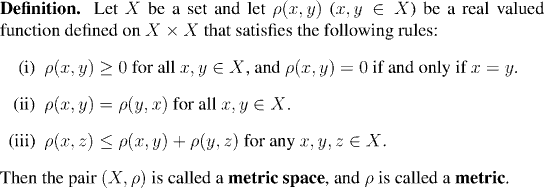We now turn to a number of examples, which relate the modes of convergence from the examples of the last chapter to metric spaces.

Example: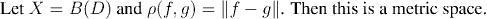Example: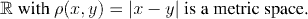Example: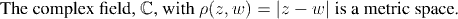Example:## Convergence and Continuity in Metric Spaces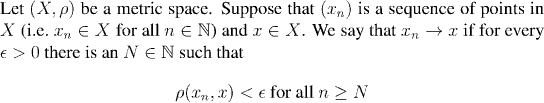The following proposition (as well as being an important fact) is a useful exercise in how to use the axioms of a metric space in proofs.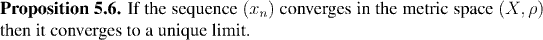Having defined convergence of sequences, we now hurry on to define continuity for functions as well. When we talk about continuity, we mean that f(x) gets close to f(y) as x gets close to y. In other words, we are measu ring the distance between both f(x) and f(y) and between x and y. So both x and f(x) are to belong to metric spaces, but there's no reason why they should belong to the same space.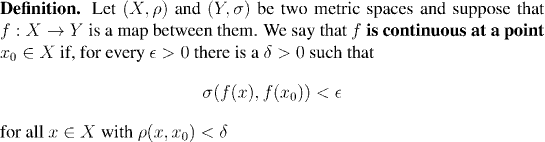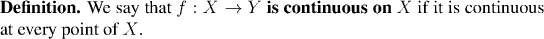We've already seen from Proposition 4.2. that it's often much more convenient to study convergence of sequences that to try to study limits or continuity of function directly. The next proposition extends that idea to continuity of functions between metric spaces.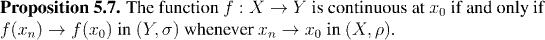The next proposition is a very useful fact for making quick justifications of why a particular sequence in a metric space converges. You'll find that it plays much the same role in studying convergence in general metric spaces, as the Sandwich Lemma did for convergence in the real numbers. In fact we already implicity used this result, at the end of the proof of Proposition 5.7, when we said "clearly" xn converges to x0 just because rho(xn, x0) is less than 1/n .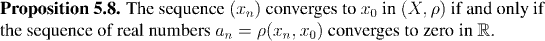The theoretical base for studying convergence and continuity is very much in line with what we did in the real numbers. When we actually get down to the nitty-gritty of proving convergence or continuity of real examples, though, the more complicated metri cs we have to work with can make things very messy. The next section examines this, and provides the tools for cutting through a lot of the mess.